# Rgraphviz test

``````## Settings for RMarkdown http://yihui.name/knitr/options#chunk_options
opts_chunk\$set(comment = "", warning = FALSE, message = FALSE, tidy = FALSE,
echo = T, fig.width = 5, fig.height = 5)
options(width = 125, scipen = 5, digits = 5)

setwd("~/statistics/Rmedstats/")
``````

## Different layout methods

``````library("Rgraphviz")
set.seed(123)
V <- letters[1:10]
M <- 1:4
g1 <- randomGraph(V, M, 0.2)

## dot
plot(g1)
``````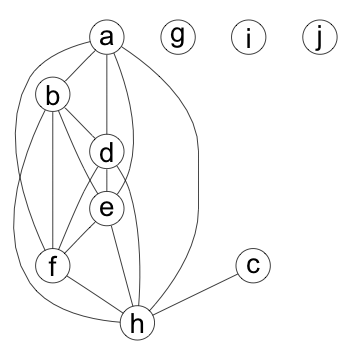``````
## neato
plot(g1, "neato")
``````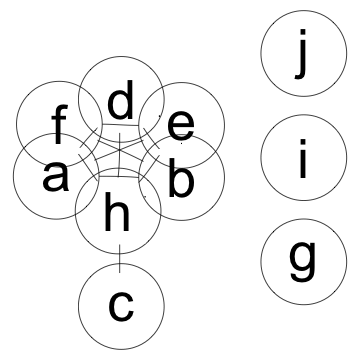``````
## twopi
plot(g1, "twopi")
``````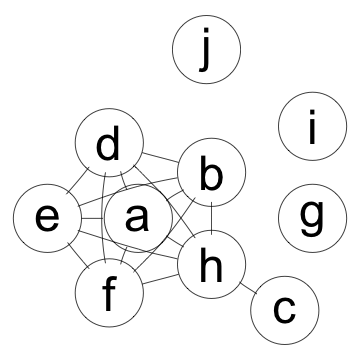## Reciprocated edges

``````rEG <- new("graphNEL", nodes = c("A", "B"), edgemode = "directed")
plot(rEG)
``````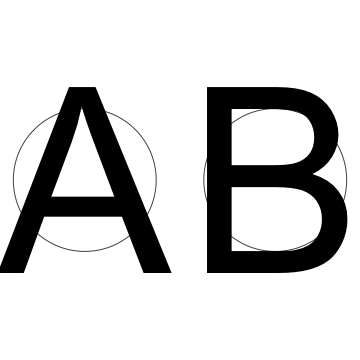``````
rEG <- addEdge("A", "B", rEG, 1)
plot(rEG)
``````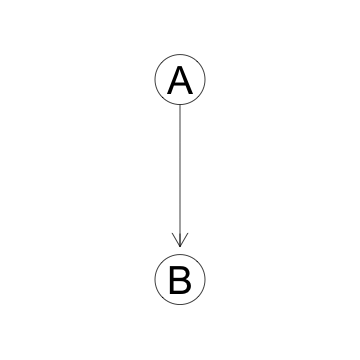``````
rEG <- addEdge("B", "A", rEG, 1)
plot(rEG)
``````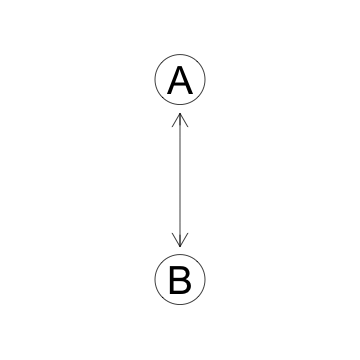``````
plot(rEG, recipEdges = "distinct")
``````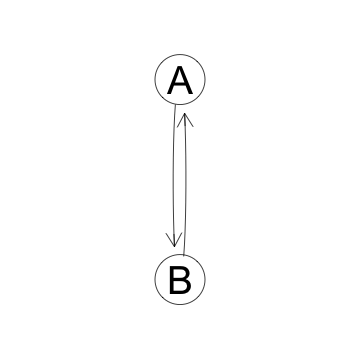## Cluster graphs

``````cG <- new("clusterGraph",
clusters = list(
a = c(1:10), b = c(11:13),
c = c(14:20), d = c(21, 22))
)
plot(cG)
``````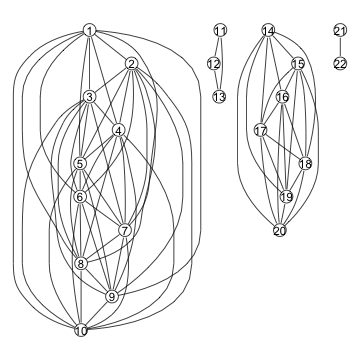## Causal diagram like

``````causal1 <- new(Class = "graphNEL", nodes = c("L", "A", "Y"), edgemode = "directed")
causal1 <- addEdge(from = "A", to = "Y", graph = causal1, weight = 2)
causal1 <- addEdge(from = "L", to = "A", graph = causal1, weight = 2)
causal1 <- addEdge(from = "L", to = "Y", graph = causal1, weight = 2)
plot(causal1)
``````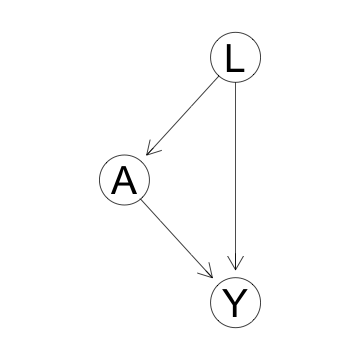causal1 <- new(Class = “track”, nodes = c(“L”, “A”, “Y”), edgemode = “directed”)
causal1 <- addEdge(from = “A”, to = “Y”, graph = causal1, weight = 2)
causal1 <- addEdge(from = “L”, to = “A”, graph = causal1, weight = 2)
causal1 <- addEdge(from = “L”, to = “Y”, graph = causal1, weight = 2)
plot(causal1)